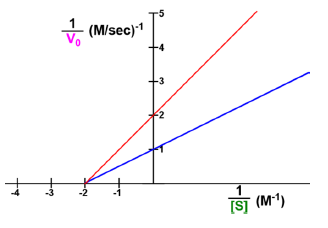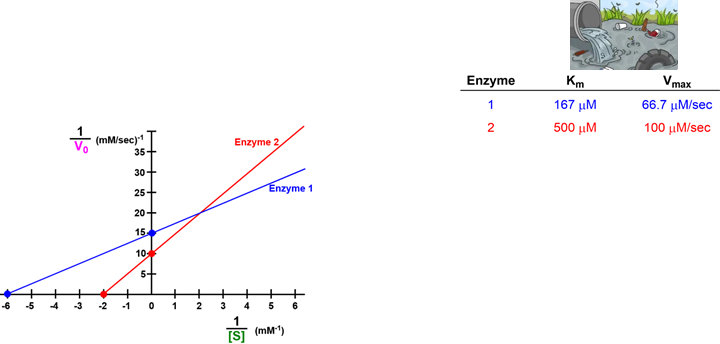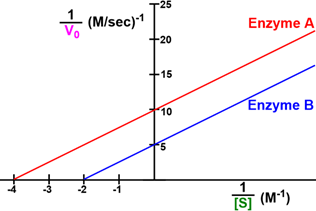Clutch Prep is now a part of Pearson
Ch. 6 - Enzymes and Enzyme KineticsWorksheetSee all chapters

# Shifting Lineweaver-Burk Plots

See all sections
Sections
Enzymes
Enzyme-Substrate Complex
Lock and Key Vs. Induced Fit Models
Optimal Enzyme Conditions
Activation Energy
Types of Enzymes
Cofactor
Catalysis
Electrostatic and Metal Ion Catalysis
Covalent Catalysis
Reaction Rate
Enzyme Kinetics
Rate Constants and Rate Law
Reaction Orders
Rate Constant Units
Initial Velocity
Vmax Enzyme
Km Enzyme
Michaelis-Menten Assumptions
Michaelis-Menten Equation
Lineweaver-Burk Plot
Michaelis-Menten vs. Lineweaver-Burk Plots
Shifting Lineweaver-Burk Plots
Calculating Vmax
Calculating Km
Kcat
Specificity Constant

Concept #1: Shifting Lineweaver-Burk Plots

Concept #2: Visualizing Increases/Decreases to Km & Vmax

Example #1: Indicate which enzyme in each graph below has a greater Km & Vmax.

Practice: Use the plot below. The Km of both enzymes for their substrate is:Practice: Suppose a company develops two enzymes that degrade highly toxic compounds to non-toxic compounds. Your task is to degrade the greatest amount of toxic compound in the shortest amount of time.

A) Which enzyme is better to use when [S] = 0.167 mM? ____________

B) Which enzyme is better to use at saturating [S]? ____________Practice: Considering the Lineweaver-Burk plot below, which of the following enzymes would be better to use (converts more substrate to product) when the [S] = 0.001 M?